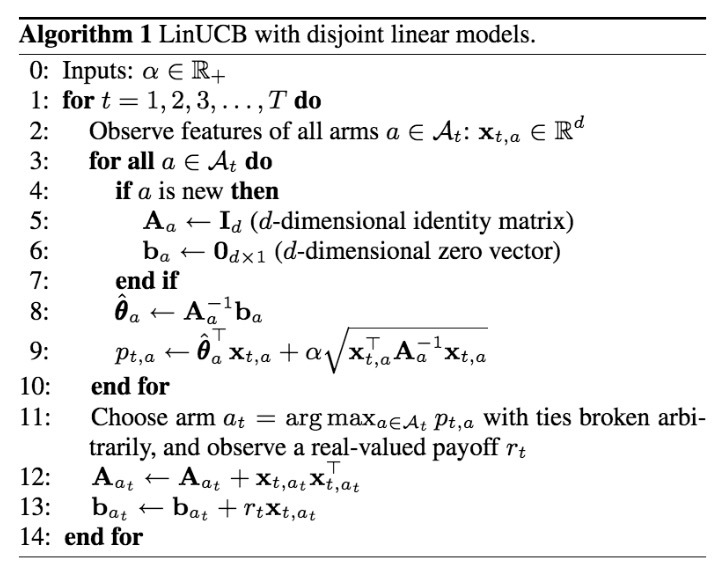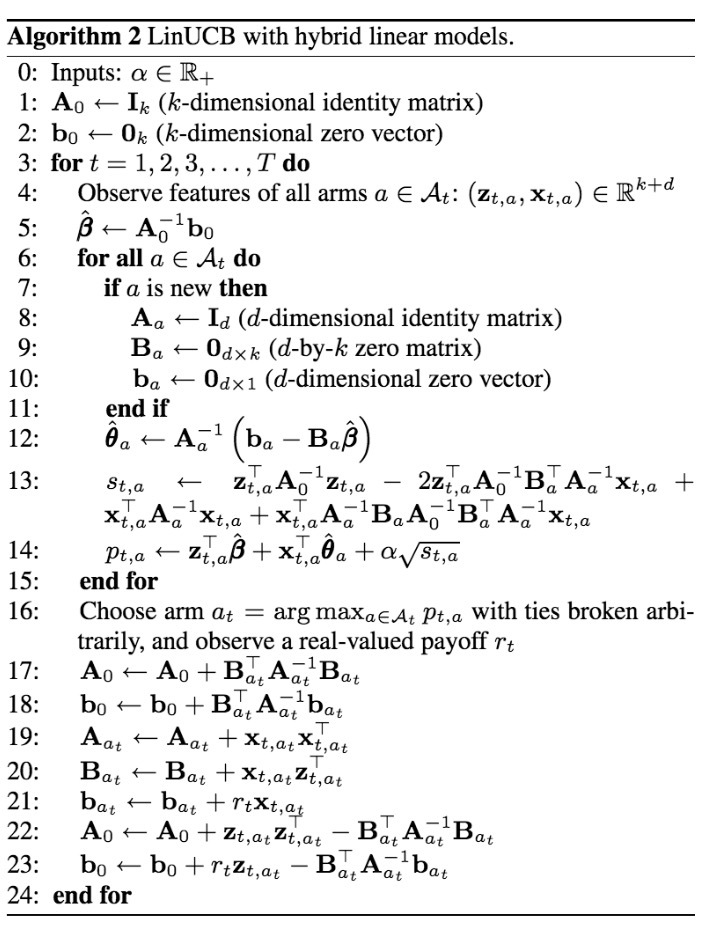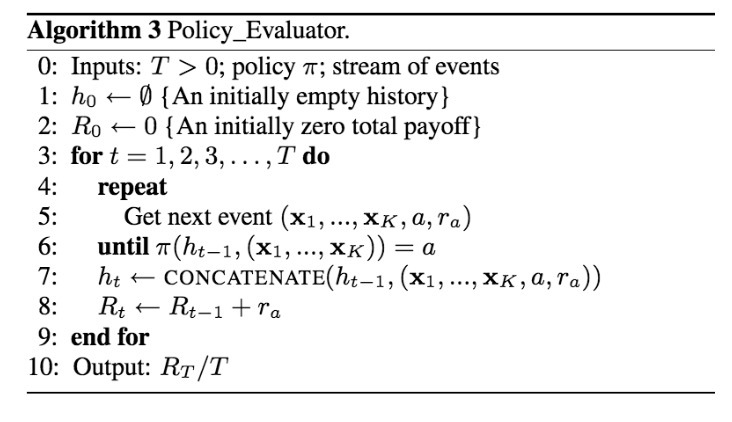# LinUCB介绍

yahoo在2010年在《A contextual-bandit approach to personalized news article recommendation》中介绍了contextual bandit算法。

# 2.公式&相关工作

## 2.1 Multi-armed Bandit公式

• 1.该算法会观察当前用户$u_t$和一个关于arms或actions的$A_t$集合，以及对于arm $a \in A_t$的它们的特征向量(feature vectors) $x_{t,a}$。 vector $x_{t,a}$会同时总结用户$u_t$和arm a的信息，被称为context
• 2.基于在之前实验中的已观察收益(observed payoffs)，算法A会选择一个arm $a_t \in A_t$，并得到新的收益(payoffs): $r_{t,a_t}$，它的期望取决于user $u_t$和arm $a_t$两者
• 3.算法接着使用新的observation $(x_{t,a_t}, a_t, r_{t,a_t})$提升它的arm-selection策略。这里需要重点强调的是：对于未选中的arms $a \neq a_t$，此处没有观察到feedback（也就是：payoff $r_{t,a}$）

$R_A(T) \equiv E[\sum\limits_{t=1}^T r_{t,a_t^*}] - E[\sum\limits_{t=1}^T r_{t,a_t}]$

…(1)

• (i) arm set $A_t$保持不变，对于所有t都包含K个arms
• (ii) user $u_t$（或者相等的，context $x_{t,1}, \cdots, x_{t,K}$）对于所有t都相同

## 2.2 已存在的Bandit算法

bandit problems的基本挑战是，需要对exploration和exploitation做平衡。为了最小化等式(1)中的regret（越小表示两者越接近），一个算法A会利用（exploits）它的过往经验来选择看起来最好的arm。另一方面，看起来最优的arm可能在实际上是次优的，因为在算法A的知识(knowledge)中是不精准的（imprecision）。为了避免这种不希望的情况，算法A必须通过实际选择看起来次优的arms来进行explore，以便收集关于它们的更多信息（在bandit过程中的step 3在之前的章节已定义）。Exploration可以增加short-term regret，因为会选到一些次优的arms。然而，获得关于arms的平均payoffs信息（例如：exploration）可以重新定义(refine)算法A的arms payoffs，从而减小long-term regret。通常，即不会存在一个纯粹的exploring，也不会存在一个纯粹的exploiting算法，需要对两者做平衡。

context-free K-armed bandit问题已经被统计学家研究过许多。一种最简单和最直接的算法是ε-greedy。在每个实验t中，该算法会首先估计每个arm a的平均payoff $\hat{\mu}_{t,a}$。接着使用概率$1 - e$来选择greedy arm（例如：具有最高payoff估计的arm）；使用概率e来选择一个random arm。在极限上，每个arm会尝试无限次，以便payoff估计$\hat{\mu_{t,a}}$会收敛到具有概率为1的真值(true value)$\mu_a$。另外，通过对e进行适当的衰减(decaying)，每一step的regret $R_A(T)/T$会收敛到0, 概率为1.

# 3.算法

## 3.1 不相交(disjoint)线性模型的LinUCB

$E[r_{t,a} | X_{t,a}] = X_{t,a}^T \theta_a^*$

…(2)

• $D_a$是在实验t上的一个m x d维的设计矩阵(design matrix)，它的行对应于m个训练输入（例如：对于文章a，之前已经观察到的m个contexts）
• $c_a \in R^m$是相应的响应向量(corresponding response vector) （例如：相应的m个 点击/未点击 user feedback） (注：paper写的是$b_a$而非$c_a$，应该是笔误)

$\hat{\theta}_a = (D_a^T D_a + I_d)^{-1} D_a^T c_a$

…(3)

$| x_{t,a}^T \hat{\theta}_a - E[r_{t,a} | x_{t,a}] | \leq \alpha \sqrt{x_{t,a}^T (D_a^T D_a + I_d)^{-1} x_{t,a}}$

…(4)

$a_t \equiv argmax_{a \in A_t} (x_{t,a}^T \hat{\theta}_a + \alpha \sqrt{x_{t,a}^T A_a^{-1} x_{t-a}})$

…(5)• 1.它的计算复杂度对于arms的数量来说是线性的，对于特征数目最多是三次方。为了减少计算量，我们可以在每一step中更新$A_{a_t}$，周期性计算和缓存$Q_a \equiv A_a^{-1}$，而非实时。
• 2.该算法对于一个动态的arm set来说工作良好，仍能高效运行，只要$A_t$的size不能太大。该case在许多应用中是true的。例如，在新闻文章推荐中，编辑会从一个池子中添加/移除文章，池子的size本质上是个常数。
• 3.尽管不会该paper的重点，我们仍会采用的分析来展示：如果arm set $A_t$是确定的，包含了K个arms，接着置信区间（比如：等式(4)的右手边）会随着越来越多的数据快速减小，接着证明$\hat{O}(\sqrt{KdT})$的强regret bound，匹配满足等式(2)的bandits的state-of-art结果。这些理论结果预示着算法的基础牢固以及高效性。

## 3.2 Hybrid线性模型的LinUCB

$E[r_{t,a} | x_{t,a}] = z_{t,a}^T \beta^* + x_{t,a}^T \theta_a^*$

…(6)

• $z_{t,a} \in R^k$是当前user/article组合的特征
• $\beta^*$是一个未知的系数向量(coefficient vector)，它对所有arms是共享的• 1.由于算法中使用的构建块（$A_0, b_0, A_a, B_a, b_a$）具有固定的维度，可以进行增量更新，该算法计算十分高效。
• 2.另外，与arms相关联的质量（quatities）在$A_t$中并不存在，因而在计算量上不再相关。
• 3.最后，我们也周期性地计算和缓存了逆($A_0^{-1}$和$A_a^{-1}$)，而非在每个实验尾部来将每个实验的计算复杂度$O(d^2 + k^2)$。

# 4.评估技术• 如果policy $\pi$选择了与logging policy所选arm的相同的arm a，那么：event仍被添加到历史中，total payoff $R_t$会被更新。
• 如果policy $\pi$选择了与logging policy所选arm所不同的arm，那么：event会被完全忽略，该算法会处理下一event，在该state上无需任何变化。

$\underset{policy\_evalutar(\pi,S)}{Pr} (h_T) = Pr_{\pi, D} (h_T)$

$\underset{policy\_evaluator(\pi,S)}{Pr} (h_{t-1}) = Pr_{\pi,D}(h_{t-1})$

$\underset{Policy\_evaluator(\pi,S)}{Pr} ((x_{t,1}, \cdots, x_{t,K}, a, r_{t,a}) | h_{t-1}) = Pr_D(x_{t,1}, \cdots, x_{t,K}, r_{t,a}) Pr_{\pi(h_{t-1})} (a | x_{t,1}, \cdots, x_{t,K})$

# 5.实验

## 5.1 Yahoo! Today模块Today模块是在Yahoo! Front Page（流量最大）的最显著位置的panel，详见图1. 在Today Module上缺省的”Featured” tab会对高质量文章（主要新闻）的1/4进行高亮（highlight）, 而4篇文章通过一个小时级别更新的由人工编辑的文章池中进行选择。如图1所示，在底部存在4篇文章，索引为F1-F4. 每篇文章由一张小图和一个标题进行表示。其中之一会在story位置进行着重展示，它由一个大图、一个标题、一个简介、以及相关链接进行着重(featured)。缺省的，在F1的文章会在story位置强调。一个用户可以点击在story位置上的highlightd文章，如果她对文章感兴趣会读取更多的详情。event被看成是一次story click。为了吸引访问者的注意力，我们想根据个人的兴趣对提供的文章进行排序，对于每个visitor在story位置上highlight最有吸引力的文章。

## 5.2 实验设置

### 5.2.1 数据收集

• (i) 提供给用户随机选中的文章
• (ii) user/article信息
• (iii) 在story位置处用户是否对该文章有点击

### 5.2.2 特征构建

• (i) 人口属性信息(demographic information)：性别（2个分类）、年龄（离散化成10个分段）
• (ii)地理特征（geographic features）：包含世界范围和美国的大约200个大都市；
• (iii)行为类别(behavioral categories)：大约1000个二分类别（binary categories），它们总结了用户在Yahoo!内的消费历史。

• (i) URL类别：数十个分类，从文章资源的URL中推断得到
• (ii) editor类别：数十个主题，由人工编辑打标签总结得到

• 我们首先通过原始的user/article features，来使用LR来拟合一个关于点击率（click probability）的bilinear model，以便$\phi_u^T W \phi_a$来近似用户u点击文章a的概率，其中$\phi_u$和$\phi_a$是相应的feature vectors，W是由LR最优化得到的权重矩阵。
• 通过计算$\psi_u = \phi_u^T W$，原始的user features接着被投影到一个induced space上。这里，用于user u，在$\psi_u$的第i个元素可以被解释成：用户喜欢文章的第i个类别的度（degree）。在induced的$\psi_u$ space中使用K-means算法将用户聚类成5个clusters。
• 最终的user feature是一个6向量（six-vector）：5个条目对应于在这5个clusters中的成员（使用一个Gaussian kernel计算，接着归一化以便他们总和一致），第6个是一个常数特征1.

## 5.3 算法比较

I.不使用特征的算法

• random: random policy总是会以等概率的方式从池子中选中候选文章之一。该算法无需参数，不会一直学习
• ε-greedy：在第2.2节所述，它会估计每篇文章的CTR；接着，它会选择具有概率e的一篇随机文章，接着选择具有概率1-e的最高CTR估计的文章。该policy只有一个参数e。
• ucb: 如2.2节所述，policy会估计每篇文章的CTR，也会估计它的置信区间，总是会选择具有最高UCB的文章。特别的，根据UCB1，我们会通过$c_{t,a} = \frac{a}{\sqrt{n_{t,a}}}$来计算一篇文章a的置信区间，其中$n_{t,a}$是在实验t之前a被选中的次数，$\alpha>0$是一个参数
• omniscient(无所不知的): 这样的一个policy会从后见之明（from hindsight）达到最好的经验型的context-free CTR。它首先会从日志事件(logged events)中计算每篇文章的经验CTR，当使用相同的logged events评估时，接着总是选择具有最高经验CTR的文章。该算法无需参数，不需要学习。

II.热启动（warm start）算法

• ε-greedy(warm):
• ucb(warm):

III.在线学习user-specific CTR的算法

# 0.介绍

Yoon Kim在《Convolutional Neural Networks for Sentence Classification》介绍了使用CNN来做句子分类的任务。下面基于对该paper的理解，简单地做个介绍：

# 1.模型架构$x_i \in R^k$ 为句子中第i个词的k维词向量。句子长度为n（不足补齐: pad），表示成：

$x_{1:n} = x_1 \oplus x_2 \oplus x_3 ... \oplus x_n$

… (1)

$\oplus$为串联操作符(concatenation operator)。 $x_{i:i+j}$ 表示 $x_i$至$x_{i+j}$的串联。

$c_i = f(w \cdot x_{i:i+h-1} + b)$

…(2)

$c = [c_1, c_2, ..., c_{n-h+1}]$

…(3)

## 2.1 Regularization

$y = w \cdot z + b$

…(4)

$y = w \cdot (z \circ r) + b$

…(5)

# 数据集

• MR: 电影评论(Movie Reviews)。分类检测正负语义。（Pang and Lee, 2005）
• SST-1: Stanford Sentiment Treebank——MR的扩展，具有train/dev/test splits，提供了细粒度标签（very positive, positive, neutral, negative, very negative）。 Socher et al. (2013)
• SST-2: 类似SST-1. 移除了neutral评论，增加了binary labels
• Subj：Subjectivity数据集，分类任务：将句子分类成：subjective or objective。(Pang and Lee, 2004).
• TREC: TREC question数据集——将一个question分类成6个问题类型（该问题是关于：person, location, numeric information, etc.） (Li and Roth, 2002)
• CR： 多种商品的顾客评价(Customer reviews)。预测positive/negative 评论。(Hu and Liu, 2004).
• MPQA：MPQA数据集的意见极性检测（Opinion polarity detection）。 (Wiebe et al., 2005).

## 3.1 超参数和训练

• ReLU
• filter window(h)为：3, 4, 5
• 每个window具有100个feature map
• dropout rate (p)为：0.5
• l2 constraint (s)为：3
• mini-batch size为：50

## 3.3 模型变种

• CNN-rand: 作为baseline模型，所有的词都是随机初始化，接着在训练中进行修改。
• CNN-static: 使用来自word2vec的pre-trained vector的model。所有的词（包括随机初始化的未登陆词）保持static，只有模型中的其它参数是通过学习得到。
• CNN-non-static: 与上面的方法相似，但对于每个任务，pre-trained vectors都会进行微调（fine-tuned）。
• CNN-multichannel: 模型具有两个词向量集合。每个向量集都看成是一个’channel’，每个filter都会作用于两个channel，但梯度的后向传播只通过其中一个channel进行。这里模型可以fine-tune一个向量集，让另一个保持static。两个channel都通过word2vec进行初始化

# 4.结果## 4.1 Multichannel vs. Single Channel Model## 4.3 进一步观察

• Kalchbrenner et al. (2014)，使用一个 CNN得到更糟的结果，本质上与single model的架构一致。例如，它们的Max-TDNN(Time Delay Neural Network)使用随机初始化的词，在SST-1上获得了37.4%，而我们的模型则为45.0%。我们将这种差异归因于：我们的CNN具有更大的容量（多个filter widths和feature maps）。
• Dropout被证明是一种很好的regularizer, 它很容易使用一个更大的网络，只需dropout去进行regularize即可。Dropout可以增加2-4%的效果提升
• 当随机初始化的词不在word2vec中时，通过从U[-a,a]中抽样每一维，可以获得微小的提升，其中选中的a，可以使随机初始化的向量具有与pre-trained vector具有相似的variance。在初始化过程，使用更复杂的方法来反映(mirror)pre-trained vectors的分布，来获得提升是挺吸引人的一件事。
• 我们试验了另一个公共的词向量（由Collobert et al. (2011) on Wikipedia训练得到），发现word2vec可以获得更好的效果提升。这一点不是很清楚：是否是因为o Mikolov et al. (2013)的架构，还是因为google news 1000亿词的数据集的原因。

# 参考

Convolutional Neural Networks for Sentence Classification

# 介绍

XGBoost的可扩展性(scalability)归因于一些重要的系统优化和算法优化。这些优化包括：

• 一种新的tree-learning算法(a novel tree learning algorithm)：用于处理稀疏数据(sparse data)
• 一种理论正确的加权分位数略图过程（a theoretically justified weighted quantile sketch procedure）：用于处理在近似的tree-learning中实例权重

• 1.设计和建立了一个高度可扩展的end-to-end tree boosting系统
• 2.提出了一种理论正确的加权分位数略图过程(theoretically justified weighted quantile sketch procedure)，用于高效地进行预计算
• 3.介绍了一种新的稀疏感知算法（sparsity-aware algorithm），用于并行化tree learning
• 4.提出了一种高效的内存感知块结构（cache-aware block structure），用于核外（out-of-core）tree learning

# 2.tree-boosting回顾

XGBoost的方法源自于Friedman的二阶方法。XGBoost在正则化目标函数上做了最小的改进。

## 2.1 正则化目标函数

$\hat{y_{i}} = \phi(x_i) = \sum_{k=1}^{K} f_k(x_i), f_k \in F$

…… (1)

$L(\phi) = \sum_{i}l(\hat{y_i}, y_i) + \sum_{i}\Omega(f_k)$

……(2)

$L^{(t)}=\sum_{i=1}^{n}l(y_i, \hat{y_i}^{(t-1)}+f_t(x_i)) + \Omega(f_t)$

$L^{(t)} \backsimeq \sum_{i=1}^{n}[l(y_i,\hat{y}^{(t-1)}) + g_i f_t(x_i) + \frac{1}{2} h_i f_t^{2}(x_i)] + \Omega(f_t)$

$L^{(t)} = \sum_{i=1}^{n} [g_i f_t(x_i) + \frac{1}{2} h_i f_t^{2}(x_i)] + \Omega(f_t)$

……(3)

$L^{(t)} = \sum_{i=1}^{n} [g_i f_t(x_i) + \frac{1}{2} h_i f_t^{2}(x_i)] + \gamma T + \frac{1}{2}\lambda\sum_{j=1}^{T}w_{j}^{2} \\ = \sum_{j=1}^{T}[(\sum_{i \in I_j} g_i)w_j + \frac{1}{2}(\sum_{i \in I_j}h_i+\lambda)w_{j}^{2}]+\gamma T$

……(4)

$w_j^{\ast}=-\frac{\sum_{i \in I_j}g_i}{\sum_{i \in I_j}h_i+\lambda}$

……(5)

$L^{(t)}(q)=-\frac{1}{2}\sum_{j=1}^{T}\frac{(\sum_{i \in I_j}g_i)^2}{\sum_{i \in I_j}h_i+\lambda} + \gamma T$

……(6)$L_{split}=\frac{1}{2}[ \frac{(\sum_{i \in I_L}g_i)^2}{\sum_{i \in I_L}h_i+\lambda} + \frac{(\sum_{i \in I_R}g_i)^2}{\sum_{i \in I_R}h_i+\lambda} - \frac{(\sum_{i \in I}g_i)^2}{\sum_{i \in I}h_i+\lambda}] - \gamma$

……(7)

# 3.Split Finding算法

## 3.1 Basic Exact Greedy Algorithm

tree learning的其中一个关键问题是，找到等式(7)的最好划分(best split)。为了达到这个目标，split finding算法会在所有特征（features）上，枚举所有可能的划分（splits）。我们称它为“完全贪婪算法(exact greedy algorithm)”。许多单机版tree-boosting实现中，包括scikit-learn，R’s gbm以及单机版的XGBoost，都支持完全贪婪算法(exact greedy algorithm)。该算法如算法1所示。它会对连续型特征（continuous features）枚举所有可能的split。为了更高效，该算法必须首先根据特征值对数据进行排序，以有序的方式访问数据来枚举等式(7)中的结构得分（structure score）的梯度统计(gradient statistics)。[算法1]

## 3.2 近似算法[算法2]## 3.3 加权分位数略图(Weighted Quantile Sketch)

$r_{k}(z)=\frac{1}{\sum_{(x,h) \in D_k} h} \sum_{(x,k) \in D_k,x<z}^{} h$

……(8)

$\|r_k(s_{k,j}) - r_k(s_{k,j+1})\| < \epsilon, s_{k1}=min_{i}^{} x_{ik}, s_{kl}=max_{i}^{} x_{ik}$

……(9)

$\sum_{i=1}^{n}\frac{1}{2}h_i(f_t(x_i)-g_i/h_i)^2 + \Omega(f_t) + constant$

## 3.4 稀疏感知的划分查找（sparsity-aware Split Finding）

• 1)数据中的missing values
• 2)统计中常见的零条目
• 3)特征工程：比如one-hot encoding[算法3]# 4.系统设计

## 4.1 用于并行学习的Column Block

tree learning最耗时的部分，是以有序方式获得数据。为了减少排序的开销，我们提出了将数据存储到内存单元（in-memory units）中，它们被称为“块（block）”。每个块中的数据，以压缩列（CSC）格式存储。每列由相应的特征值进行排序。输入数据的布局，在训练前只需要计算一次，在后续迭代中可复用。d为树的最大深度，K为树的总树目。对于exact greedy algorithm，原始的稀疏感知算法的时间复杂度：

$O(K d |x|_{0}logn)$

$O(Kd {|x|} _0 + {|x|}_{0}logn)$

$O(K d {|x|}_{0} log q)$

$O(K d{|x|}_{0} + {|x|}_{0} logB)$

## 4.2 内存感知访问（Cache-aware Access）## 4.3 Out-of-core计算# 参考

XGBoost: A Scalable Tree Boosting System

# coec介绍

microsoft在《Position-Normalized Click Prediction in Search Advertising》对coec做了介绍。

# 2.因子模型

• c表示点击次数
• v表示曝光次数

$p(click | i,j) = p(click | exam, i) p(exam | j)$

…(1)

• 第一个因子 $p(click \mid exam, i)$：可以简单表示为$p_i$，它是一个位置归一化的CTR（position-normalized CTR），可以表示ad的相关度
• 第二个因子 $p(exam \mid j)$，可以简单表示为$q_j$，体现了位置偏差（ positional bias）。

# 3. Binomial模型

$c_{ij} \sim Binomial(v_{ij}, p_i q_j), \forall i,j$ 。。。

# 4.POISSON模型

$c_{ij} \sim Poisson(v_{ij} p_i q_j), \forall i,j$

…(9)

# 5.GAMMA-POISSON模型

$q_j \sim Gamma(\alpha, \beta), \forall j$

…(16)

。。。

# 7.实验

## 7.1 使用人造数据仿真

• 1.当从真实噪声中进行抽象时，允许快速研究大量参数
• 2.通过该数据曝露的真实分布来验证学到的模型，对于真实数据很重要

1. $\foreach$ 位置 $position j \in [1,...,m]$，生成一个$q_j \sim Gamma(\alpha,\beta)$，以降序对q排序，通过$1/q_1$对q进行缩放
• 2.

# LightFM介绍

lightFM源自于paper: 《Metadata Embeddings for User and Item Cold-start》：

# 一、介绍

• 1.在冷启动和低密度场景，LightFM至少与纯CB模型一样好，实质上，当满足以下二者之一（1）在训练集中提供了协同信息 (2) 在模型中包含了用户特征 时，效果要更好。
• 2.当协同数据很丰富时（warm-start, dense user-item matrix），LightFM至少与MF模型效果一样好。
• 3.通过LightFM生成的Embeddings，可以编码关于features的重要语义信息，可以被用于相关推荐任务：比如：标签推荐。

LightFM python版的Github地址为：https://github.com/lyst/lightfm.

# 2.LightFM

## 2.1 动机

LightFM模型的结构受以下两种考虑的启发：

• 1.该模型必须能从交互数据中学习user和item表示：如果描述为“舞会袍（ball gown）”和”铅笔裙(pencil skirt)”的items均被用户所喜欢，该模型必须能学到ball gowns与pencil skirts相似。
• 2.该模型必须能为新items和users计算推荐

## 2.2 模型

Users和items通过它们的features进行完全描述。每个user u通过一个特征集合描述 $f_u \subset F^U$。为每个item i它们的特征为$f_i \subset F^I$。features是提前知道的，可以表示user和item的metadata。

user u的隐表示，通过对它的features的隐向量进行求和来表示：

$q_u = \sum_{j \in f_u} e_j^U$

item i的隐表示类似，如下：

$p_i = \sum_{j \in f_i} e_j^I$

user u的bias项，通过对features的biases进行求和得到：

$b_u = \sum_{j \in f_u} b_j^U$

item i的bias项如下：

$b_i = \sum_{j \in f_i} b_j^I$

$\hat{r_{ui}} = f(q_u \cdot p_i + b_u + b_i)$

…(1)

$f(x)= \frac{1} {1 + exp(-x)}$

$L(e^U, e^I, b^U, b^I) = \prod_{(u,i)\in S^+} \hat{r_{ui}} \times \prod_{(u,i)\in S^-} (1-\hat{r_{ui}}$

…(2)